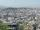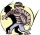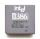A radioactive material loses 10% of its mass each year. What proportion will be left there after n=6 years?

Result

x =  0.531

Solution:Leave us a comment of example and its solution (i.e. if it is still somewhat unclear...):Be the first to comment!Next similar examples:

1. Investment1000\$ is invested at 10% compound interest. What factor is the capital multiplied by each year? How much will be there after n=12 years?
2. The cityAt the end of 2010 the city had 248000 residents. The population increased by 2.5% each year. What is the population at the end of 2013?
3. The crimeThe crime rate of a certain city is increasing by exactly 7% each year. If there were 600 crimes in the year 1990 and the crime rate remains constant each year, determine the approximate number of crimes in the year 2025.
4. Computer revolutionWhen we started playing with computers, the first processor, which I remember was the Intel 8080 from 1974, with the performance of 0.5 MIPS. Calculate how much percent a year rose CPU performance when Intel 486DX from 1992 has 54 MIPS. What
5. Account operationsMy savings of php 90,000 in a bank earns 6% interest in a year. If i will deposit additional php 10,000 at the end of 6 months, how much money will be left if i withdraw php 25,000 after a year?
6. Annual pensionCalculate the amount of money generating an annual pension of EUR 1000, payable at the end of the year and for a period of 10 years, shall be inserted into the bank to account with an annual interest rate of 2%
7. DemographicsThe population grew in the city in 10 years from 42000 to 54500. What is the average annual percentage increase of population?
8. A perineumA perineum string is 10% shorter than its original string. The first string is 24, what is the 9th string or term?
9. Theorem proveWe want to prove the sentence: If the natural number n is divisible by six, then n is divisible by three. From what assumption we started?
10. SequenceCalculate what member of the sequence specified by ? has value 86.
11. The city 2Today lives 167000 citizens in city. How many citizens can we expect in 11 years if their annual increase is 1%?
12. Tenth memberCalculate the tenth member of geometric sequence when given: a1=1/2 and q=2
13. Five membersWrite first 5 members geometric sequence and determine whether it is increasing or decreasing: a1 = 3 q = -2
14. Six termsFind the first six terms of the sequence a1 = -3, an = 2 * an-1
15. GP - 8 itemsDetermine the first eight members of a geometric progression if a9=512, q=2
16. Geometric sequence 4It is given geometric sequence a3 = 7 and a12 = 3. Calculate s23 (= sum of the first 23 members of the sequence).
17. Geometric progression 2There is geometric sequence with a1=5.7 and quotient q=-2.5. Calculate a17.